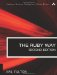# Section 5.4. Comparing Floating Point Numbers

### 5.3. Rounding Floating Point Values

Kirk: What would you say the odds are on our getting out of here?

Spock: It is difficult to be precise, Captain. I should say approximately 7824.7 to one.

Star Trek, "Errand of Mercy"

If you want to round a floating point value to an integer, the method round will do the trick:

`pi = 3.14159 new_pi = pi.round   # 3 temp = -47.6 temp2 = temp.round  # -48`

Sometimes we want to round not to an integer but to a specific number of decimal places. In this case, we could use sprintf (which knows how to round) and eval to do this:

`pi = 3.1415926535 pi6 = eval(sprintf("%8.6f",pi))  # 3.141593 pi5 = eval(sprintf("%8.5f",pi))  # 3.14159 pi4 = eval(sprintf("%8.4f",pi))  # 3.1416`

Of course, this is somewhat ugly. Let's encapsulate this behavior in a method that we'll add to Float:

`class Float   def roundf(places)     temp = self.to_s.length     sprintf("%#{temp}.#{places}f",self).to_f   end end`

Occasionally we follow a different rule in rounding to integers. The tradition of rounding n+0.5 upward results in slight inaccuracies at times; after all, n+0.5 is no closer to n+1 than it is to n. So there is an alternative tradition that rounds to the nearest even number in the case of 0.5 as a fractional part. If we wanted to do this, we might extend the Float class with a method of our own called round2, as shown here:

`class Float   def round2     whole = self.floor     fraction = self - whole     if fraction == 0.5       if (whole % 2) == 0         whole       else         whole+1       end     else       self.round     end   end end a = (33.4).round2  # 33 b = (33.5).round2  # 34 c = (33.6).round2  # 34 d = (34.4).round2  # 34 e = (34.5).round2  # 34 f = (34.6).round2  # 35`

Obviously round2 differs from round only when the fractional part is exactly 0.5; note that 0.5 can be represented perfectly in binary, by the way. What is less obvious is that this method works fine for negative numbers also. (Try it.) Also note that the parentheses used here are not actually necessary but are used for readability.

Now, what if we wanted to round to a number of decimal places, but we wanted to use the "even rounding" method? In this case, we could add a method called roundf2 to Float:

`class Float   # round2 definition as before   def roundf2(places)     shift = 10**places     (self * shift).round2 / shift.to_f   end end a = 6.125 b = 6.135 x = a.roundf2(a)   # 6.12 y = b.roundf2(b)   # 6.13`

The preceding code (roundf and roundf2) has certain limitations, in that a large floating point number naturally causes problems when it is multiplied by a large power of ten. For these occurrences, error-checking should be added.The Ruby Way, Second Edition: Solutions and Techniques in Ruby Programming (2nd Edition)
ISBN: 0672328844
EAN: 2147483647
Year: 2004
Pages: 269
Authors: Hal Fulton

Similar book on Amazon

flylib.com © 2008-2017.
If you may any questions please contact us: flylib@qtcs.net